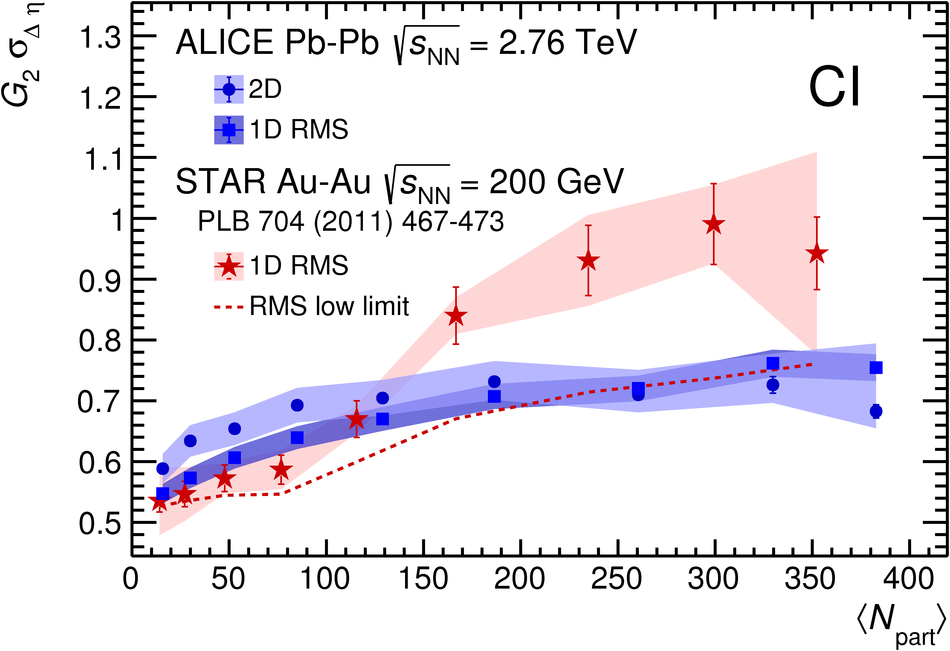# Figure 4

 Two-particle transverse momentum correlation $G_{2}^{\rm{CI}}$ longitudinal width evolution with the number of participants in Au--Au collisions at $\sqrt{s_{\rm{NN}}}=200\;\text{\GeVe}$~ and in Pb--Pb collisions at $\sqrt{s_{\rm{NN}}}=2.76\;\text{\TeVe}$, measured in this work, using the bi-dimensional fit described in the text (2D) and the method used by the STAR experiment~~(1D). For completeness, STAR RMS low limit~ is also shown.